Next: The optimal weight g Up: Parametric and zone indexes Previous: Weighted zone scoring   Contents   Index

## Learning weights

How do we determine the weights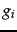for weighted zone scoring? These weights could be specified by an expert (or, in principle, the user); but increasingly, these weights are learned'' using training examples that have been judged editorially. This latter methodology falls under a general class of approaches to scoring and ranking in information retrieval, known as machine-learned relevance . We provide a brief introduction to this topic here because weighted zone scoring presents a clean setting for introducing it; a complete development demands an understanding of machine learning and is deferred to Chapter 15 .

1. We are provided with a set of training examples, each of which is a tuple consisting of a query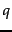and a document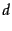, together with a relevance judgment foron. In the simplest form, each relevance judgments is either Relevant or Non-relevant. More sophisticated implementations of the methodology make use of more nuanced judgments.
2. The weightsare then learned'' from these examples, in order that the learned scores approximate the relevance judgments in the training examples.

For weighted zone scoring, the process may be viewed as learning a linear function of the Boolean match scores contributed by the various zones. The expensive component of this methodology is the labor-intensive assembly of user-generated relevance judgments from which to learn the weights, especially in a collection that changes frequently (such as the Web). We now detail a simple example that illustrates how we can reduce the problem of learning the weightsto a simple optimization problem.

We now consider a simple case of weighted zone scoring, where each document has a title zone and a body zone. Given a queryand a document, we use the given Boolean match function to compute Boolean variables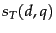and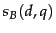, depending on whether the title (respectively, body) zone ofmatches query. For instance, the algorithm in Figure 6.4 uses an AND of the query terms for this Boolean function. We will compute a score between 0 and 1 for each (document, query) pair usingandby using a constant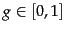, as follows: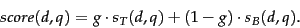(14)

We now describe how to determine the constant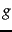from a set of training examples, each of which is a triple of the form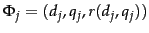. In each training example, a given training document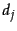and a given training query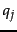are assessed by a human editor who delivers a relevance judgment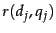that is either Relevant or Non-relevant. This is illustrated in Figure 6.5 , where seven training examples are shown.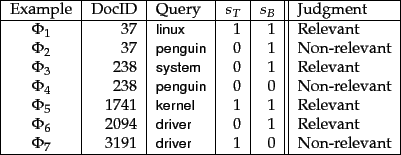For each training example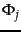we have Boolean values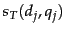and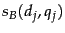that we use to compute a score from (14)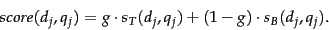(15)

We now compare this computed score to the human relevance judgment for the same document-query pair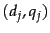; to this end, we will quantize each Relevant judgment as a 1 and each Non-relevant judgment as a 0. Suppose that we define the error of the scoring function with weightas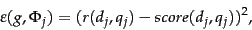(16)

where we have quantized the editorial relevance judgmentto 0 or 1. Then, the total error of a set of training examples is given by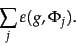(17)

The problem of learning the constantfrom the given training examples then reduces to picking the value ofthat minimizes the total error in (17).

Picking the best value ofin (17) in the formulation of Section 6.1.3 reduces to the problem of minimizing a quadratic function ofover the interval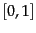. This reduction is detailed in Section 6.1.3 .Next: The optimal weight g Up: Parametric and zone indexes Previous: Weighted zone scoring   Contents   Index